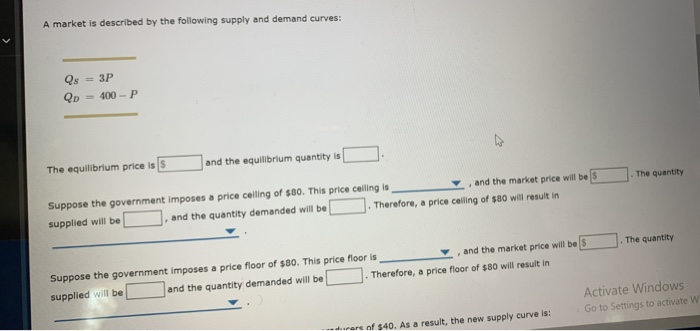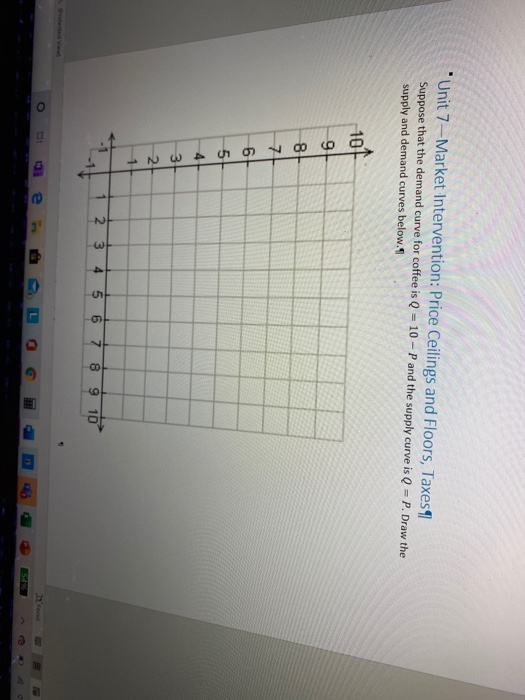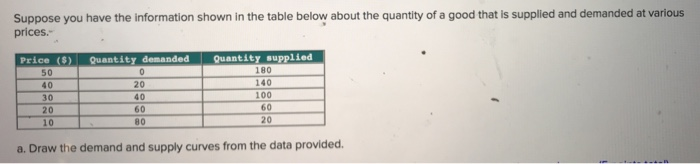Question

# Fill in the blanks below with decimal numbers (not fractions): Suppose the demand for a product...

Fill in the blanks below with decimal numbers (not fractions):

Suppose the demand for a product can be modeled with the equation P = 100 − 2Q and the corresponding supply curve can be modeled with P = 3Q.

In equilibrium, _____________units of the good will be sold at a price of \$ ________each.

At \$66, the quantity demanded is __________units.

At \$51, the quantity supplied is ________units

The deadweight loss of a \$66 price floor would be \$_________

In equilibrium, quantity demanded is equal to the quantity supplied so that we have

100-2Q=3Q

Q=100/5= 20 units. Price= 3*20 = \$60

Therefore in equilibrium 20 units of the good will be sold at a price of \$60.

When the price is \$66 quantity demanded is 17 units

When the price is \$51 the quantity supplied is 17 units.

At a price floor of 66 dollar, quantity demanded is 17 units and quantity supplied is 22 units.

Deadweight loss is given by 0.5*(66-51)*(20-17) = 22.50

#### Earn Coins

Coins can be redeemed for fabulous gifts.

Similar Homework Help Questions
• ### Suppose the demand for a good is modeled by the equation Q = 5 - 3P,...

Suppose the demand for a good is modeled by the equation Q = 5 - 3P, and the supply for the good is modeled by Q = 2 + P. Fill in the blanks below with decimal answers (i.e. no fractions). The equilibrium quantity of the good will be ________ and the equilibrium price will be ________.

• ### A market is described by the following supply and demand curves: Qs = 3P Qd =...A market is described by the following supply and demand curves: Qs = 3P Qd = 400-P The equilibrium price is S and the equilibrium quantity is Suppose the government imposes a price ceiling of \$80. This price ceiling is , and the market price will be supplied will be . and the quantity demanded will be . Therefore, a price calling of \$60 will result in the quantity the quantity Suppose the government imposes a price floor of \$80....

• ### not sure what equation to use to find the supply and demand curves Unit 7-Market Intervention:...not sure what equation to use to find the supply and demand curves Unit 7-Market Intervention: Price Ceilings and Floors, Taxes Suppose that the demand curve for coffee is Q = 10-P and the supply curve is Q = P. Draw the supply and demand curves below. NWU1000 2 3 4 5 6 7 8 9 10 1. What is the equilibrium price and quantity? 2. What is total surplus, consumer surplus, and producer surplus? 3. Suppose the government implemented...

• ### 10. Problems and Applications Q10 A market is described by the following supply and demand curves:...

10. Problems and Applications Q10 A market is described by the following supply and demand curves: QS = 4P QD = 400-P The equilibrium price is \$_______  and the equilibrium quantity is _______ . Suppose the government imposes a price ceiling of \$90. This price ceiling is _______ , and the market price will be \$_______ . The quantity supplied will be _______ and the quantity demanded will be _______ . Therefore, a price ceiling of \$90 will result in _______ . Suppose the government imposes a price...

• ### Suppose you have the information shown in the table below about the quantity of a good...Suppose you have the information shown in the table below about the quantity of a good that is supplied and demanded at various prices. Price (S) Quantity demanded Quantity supplied 50 40 30 20 10 20 40 60 80 180 140 100 60 20 a. Draw the demand and supply curves from the data provided. Instructions: Round your answers to the nearest whole number and include a negative sign if appropriate. b. The equilibrium price is \$. and the equilibrium...

• ### 10. Problems and Applications Q10 A market is described by the following supply-and-demand curves: QSQS =  =...

10. Problems and Applications Q10 A market is described by the following supply-and-demand curves: QSQS =  = 3P3P QDQD =  = 400−P400−P The equilibrium price is and the equilibrium quantity is .Suppose the government imposes a price ceiling of \$120. This price ceiling is   , and the market price will be . The quantity supplied will be , and the quantity demanded will be . Therefore, a price ceiling of \$120 will result in   .Suppose the government imposes a price floor of...

• ### The market for rice in a country has the following demand and supply functions: Demand function:                     ...

The market for rice in a country has the following demand and supply functions: Demand function:                      P = 6 – 0.5QD Supply function:         P = 2 + 0.5QS Where QD is the quantity demanded, QS is the quantity supplied and P is the unit price of rice. Determine the equilibrium price, quantity, consumer surplus and producer surplus in the rice market. Illustrate your answers with a suitable rice market diagram. (8 marks) To help the rice farmers, the government has...

• ### Suppose demand and supply are given by Qd = 50 - P and Qs  = 0.5P -...

Suppose demand and supply are given by Qd = 50 - P and Qs  = 0.5P - 10. a. What are the equilibrium quantity and price in this market? Equilibrium quantity: Equilibrium price: b. Determine the quantity demanded, the quantity supplied, and the magnitude of the surplus if a price floor of \$48 is imposed in this market. Quantity demanded: Quantity supplied: Surplus: c. Determine the quantity demanded, the quantity supplied, and the magnitude of the shortage if a price ceiling...

• ### For Question 1-8, consider a competitive market for a good where the demand curve is determined...

For Question 1-8, consider a competitive market for a good where the demand curve is determined by the demand function: P=5-QD and the supply curve is determined by the supply function: P=QS. Where P stands for Price, QD is quantity demanded and QS is quantity supplied. What is the equilibrium price level for the good in the competitive market?

• ### Suppose that the demand curve for organic tomatoes is Q = 120-10p, and the supply curve...

Suppose that the demand curve for organic tomatoes is Q = 120-10p, and the supply curve is Q=10p. The government imposes a price control of p = 4. (a) Without government intervention, what is the equilibrium price and quantity? (b) Without government intervention, what is the consumer surplus, producer surplus, and deadweight loss? Use a graph in your calculations. (c) Is the price control a price ceiling or price floor? Why? With the price control, what is the new equilibrium...